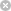RTXI  2.1learncur.cpp
Go to the documentation of this file.
1 //
2 // File = learncur.cpp
3 //
4
5 #include <iostream>
6 #include <fstream>
7 #include <stdlib.h>
8
9 #include "learncur.h"
10 #ifdef _DEBUG
11 extern std::ofstream DebugFile;
12 #endif
13
14 using namespace std;
15
16 LearningCurve::LearningCurve(int num_samps, int num_trials, double min_mse)
17 {
18  Num_Samps = num_samps;
19  Num_Trials = num_trials;
20  Min_Mse = min_mse;
21  Buffer = new double[Num_Samps];
22  Sqr_Buf = new double[Num_Samps];
23  Trial_Buf = new double[Num_Samps];
24  for (int i = 0; i < num_samps; i++) {
25  Buffer[i] = 0.0;
26  Sqr_Buf[i] = 0.0;
27  Trial_Buf[i] = 0.0;
28  }
29 }
30 void
32  LOGICAL_T save_this_trial)
33 {
34  double sqr_err;
35  sqr_err = err_samp * err_samp;
36  Buffer[samp_idx] += sqr_err;
37  Sqr_Buf[samp_idx] += sqr_err * sqr_err;
38  if (save_this_trial == _TRUE)
39  Trial_Buf[samp_idx] = sqr_err;
40 }
41 void
43 {
44  double mean, var;
45  ofstream out_file("learncur.txt", ios::out);
46  for (int i = 0; i < Num_Samps; i++) {
47  // mean = Buffer[i]/(Num_Trials-1);
48  // var = (Sqr_Buf[i]/(Num_Trials-1)) - (mean*mean);
49  mean = Buffer[i] / Num_Trials;
50  var = (Sqr_Buf[i] / Num_Trials) - (mean * mean);
51  mean = Buffer[i] / Num_Trials;
52  /*
53  out_file << i << ", "
54  << (mean-Min_Mse) << ", "
55  << (Trial_Buf[i]-Min_Mse) << std::endl;
56  */
57
58  out_file << i << ", " << mean << ", " << Trial_Buf[i] << ", " << Min_Mse
59  << std::endl;
60  }
61  out_file.close();
62 }
void AddSample(int samp_idx, double err_samp, LOGICAL_T save_this_trial)
Definition: learncur.cpp:31
void DumpCurve(void)
Definition: learncur.cpp:42
LOGICAL_T
Definition: typedefs.h:7
Definition: typedefs.h:9
LearningCurve(int num_samps, int num_trials, double min_mse)
Definition: learncur.cpp:16
std::ofstream DebugFile